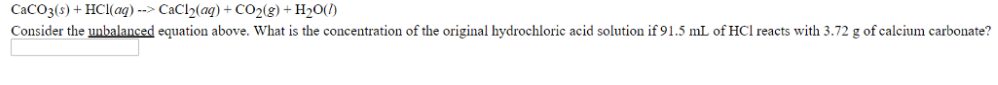# Problem: CaCO3 (s) + HCl (aq) → CaCl2 (aq) + CO2 (g) + H2O (l)Consider the unbalanced equation above. What is the concentration of the original hydrochloric acid solution if 91.5 mL of HCl reacts with 3.72 g of calcium carbonate?

###### FREE Expert Solution
83% (302 ratings)###### Problem Details

CaCO3 (s) + HCl (aq) → CaCl2 (aq) + CO2 (g) + H2O (l)

Consider the unbalanced equation above. What is the concentration of the original hydrochloric acid solution if 91.5 mL of HCl reacts with 3.72 g of calcium carbonate?